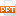# Tài chính doanh nghiệp - Chapter 7: Interest rates and bond valuation

How about another one? Assume you have the following information. Seagrams bonds have a \$1000 face value The promised annual coupon is \$100 The bonds mature in 20 years The market’s required return on similar bonds is 12% 1. Calculate the present value of the face value = \$1000  [1/1.1220 ] = \$1000  .10366 = \$103.66 2. Calculate the present value of the coupon payments = \$100  [1 - (1/1.1020)]/.10 = \$100  7.4694 = \$746.94 3. The value of each bond = \$103.66 + 746.94 = \$850.60 Why is this bond selling at a discount to its face value?39 trang | Chia sẻ: thuychi20 | Ngày: 07/04/2020 | Lượt xem: 59 | Lượt tải: 0
Bạn đang xem trước 20 trang tài liệu Tài chính doanh nghiệp - Chapter 7: Interest rates and bond valuation, để xem tài liệu hoàn chỉnh bạn click vào nút DOWNLOAD ở trên
•chap007_0292.ppt